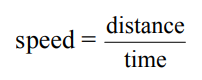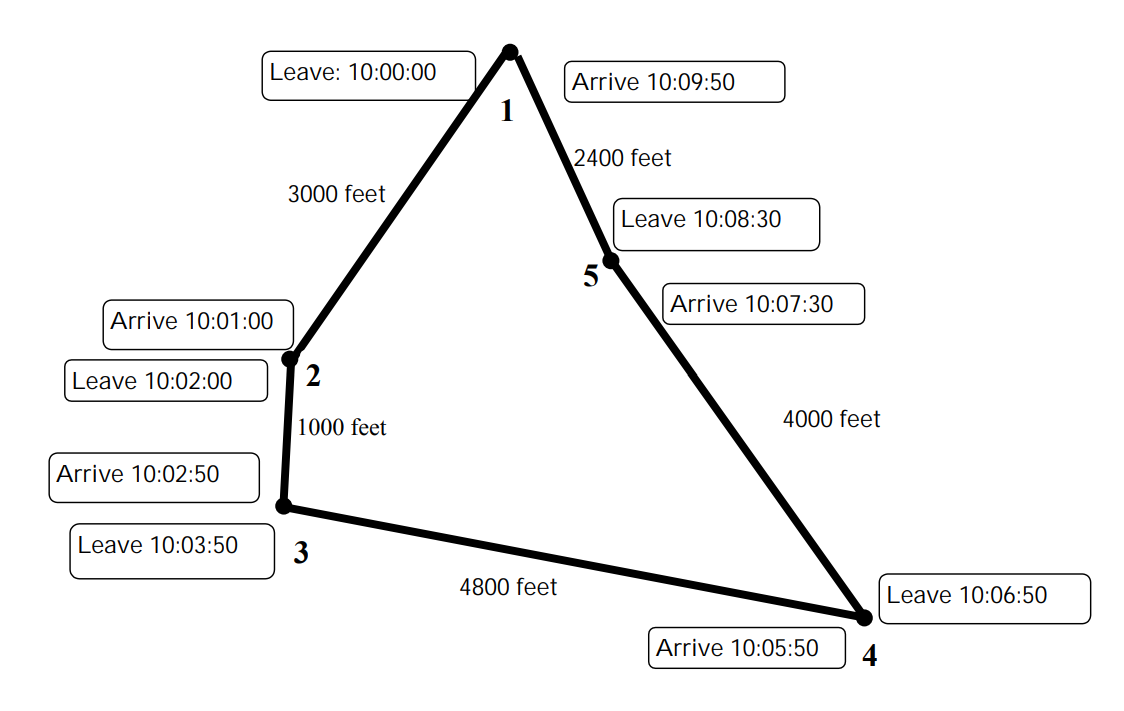Subject:
Mathematics

Topics: Measurement (general)
Common Core State Standard: 4.MD.2,
Concepts:

Relationship between distance, time and speed.

Knowledge and Skills:

Division, determining speeds

Lesson:

Procedure:

Students can work on this activity individually or in teams or two. If you feel that your students will need help to work out an approach to solving this problem, you may wish to get them started as follows:

Start by asking students to tell you what it is that they are being asked to determine (where the train goes the fastest). Then ask them what information they would need to have to answer that question (the speed of the train for each part of its route.)

Next ask what information they would need to know to determine the speed (distance and time). (If they have not already learned the speed formula, then you should review that formula with them now.)

Write the formula on the board:Ask how they could find the distance from point 1 to point 2 (it is labeled on the map, 3000 feet).

Ask how they could determine the time taken. Discuss until all students understand that they can find the time by subtracting the time when the train leaves point 1 from the time when the train arrives at point 2. Tell students that they should all use “seconds” as the time unit in this activity, and so the time from point 1 to point 2 is 60 seconds.

Then have the students use the formula to determine the speed:

speed = 3000 feet / 60 seconds
3000 ÷ 60 = 50
speed = 50 feet per second

Ask students to work in teams to determine the speed for the other parts of the train’s route. While they are doing so, circulate and help the teams work through any difficulties.

Point 1 to point 2: Distance = 3000 feet, time = 60 seconds, speed = 50 feet per second.

Point 2 to point 3: Distance = 1000 feet, time = 50 seconds, speed = 20 feet per second.

Point 3 to point 4: Distance = 4800 feet, time = 120 seconds, speed = 40 feet per second.

Point 4 to point 5: Distance = 4000 feet, time = 40 seconds, speed = 100 feet per second.

Point 5 to point 1: Distance = 2400 feet, time = 80 seconds, speed = 30 feet per second.

### Subway Speed

This is a picture of a subway route. Between what two points does the subway train travel the fastest?